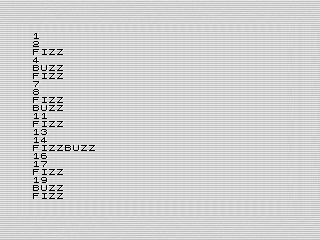# Accelerating FizzBuzz on the ZX81: A Basic Guide to High-Speed Integer Printing

Revisiting my FizzBuzz reduce the delay when printing numbers..

Reading some old programming books from the eighties, I saw that the ZX81 had an `ABS` command that made me think I could improve my FizzBuzz program. It was late and my mind mixed that command with a modulus one, which the ZX81 doesn’t have. In any case, it offered me an excuse to fix the latency introduced by printing numbers.

# A first attempt.

Thinking I could improve a bit from my previous attempt using a fast string array. For FizzBuzz, I started by creating a more generic version of that routine. I wanted to see if I could get rid of needing the digits array. The advantage of this routine is that I could print any three digit number—or larger if you change starting DIGIT variable. After some troubleshooting, I was able to get the routine working.

` 300 REM **PRINT NUMBER** 310 LET DIGIT=100 315 LET TEMP=I 316 LET LEAD=10 320 IF TEMP<DIGIT THEN GOTO 360 325 LET LEAD=0 330 LET NUM=INT (TEMP/DIGIT) 340 PRINT "0123456789"(NUM+1); 350 LET TEMP=TEMP-NUM*DIGIT 360 LET DIGIT=DIGIT/10 370 IF DIGIT<1 THEN RETURN 380 GOTO 330-LEAD`

The biggest advantage of this version over the former was that it avoided printing leading zeros. Although faster than printing actual numbers, it wasn’t a noticeable improvement. Worse, the math itself is slow enough to add a lag between each digit printed. It is quite obvious and wasn’t my intent. Thus, although it works, it isn't what I wanted.

# Going back and taking a different approach.

Being disappointed, I went back to my original routine with much simpler math. Since the loop just increments by one, I integrated the routine into the loop. I was able to rearrange a few things to create an initialization routine (lines 250–290) before the first increment. Thus counting began at one, which is where FizzBuzz started at.

` 250 LET N\$="0123456789" 260 DIM D(3) 280 LET T\$="PI*PI+1" 290 LET Z\$="NOT PI" 300 LET D(1)=D(1)+1 310 IF D(1)<11 THEN RETURN 320 LET D(2)=D(2)+1+(1 AND NOT D(2)) 330 LET D(1)=1 340 IF D(2)<11 THEN RETURN 350 LET D(3)=D(3)+1+(1 AND NOT D(3)) 360 LET D(2)=1 370 RETURN`

In the code above, the T\$ and Z\$ are from the original FizzBuzz program and added a bit of delay. The rest of the code mimicked the original fast number printing routine. As noted before, the digits are offset by one to deal with the ZX81’s string arrays starting at 1. On this occasion, this behavior works to my advantage.

Since I still wanted to avoid printing leading zero’s, I could add a check to avoid that if the array counter is at zero or an invalid number. Instead of printing all the digits, I turned the print number routine into a series of checks to avoid printing unwanted digits. With those changes, and to my surprise, the final routine removed much of the earlier lag. Here is the code to print the number:

` 400 IF D(3) THEN PRINT N\$(D(3)); 410 IF D(2) THEN PRINT N\$(D(2)); 420 PRINT N\$(D(1)); 430 RETURN`

With that, the program behaves as it did before, but was a bit faster without any noticeable delay.FizzBuzz 2, ZX81 Screenshot, 2023 by Steven Reid

# Finishing up.

All that was left was to clean up the code, which consisted mostly of reordering the line numbers. The above snippets are from the final version. But the result is exactly as I wanted. Whether a number, fizz, buzz or fizz buzz, the program prints each quickly without any lag. The new flow is exactly what I was trying for and the code is still relatively readable. Success!